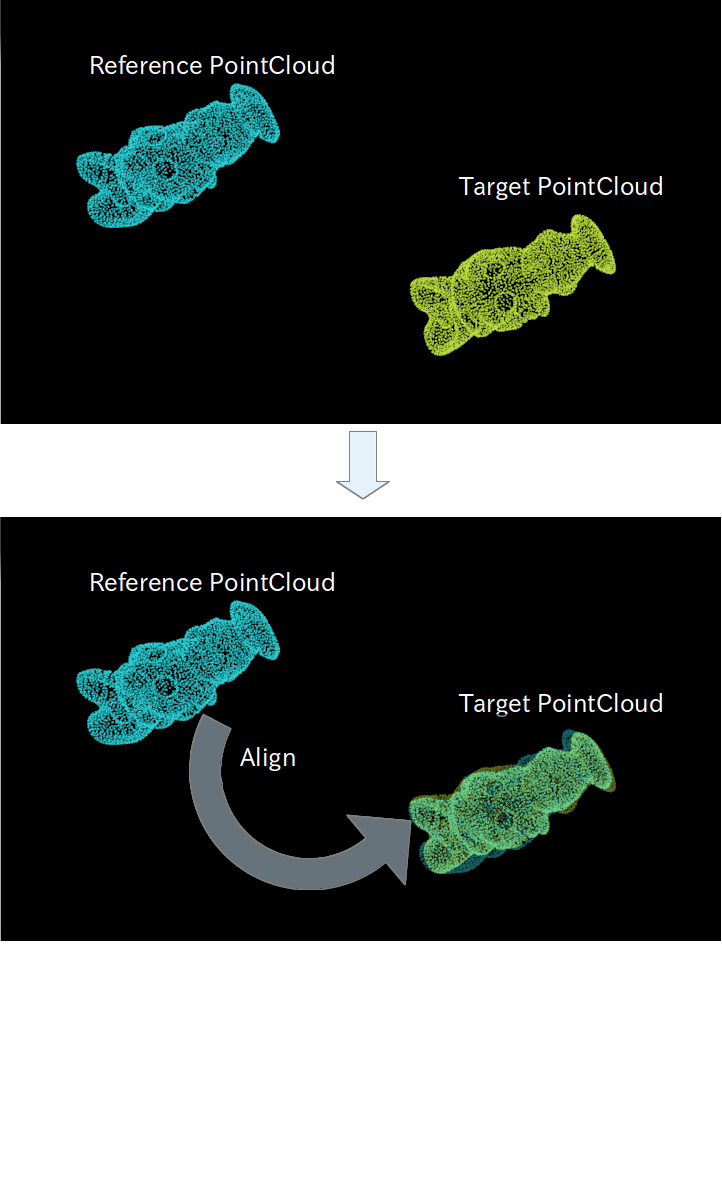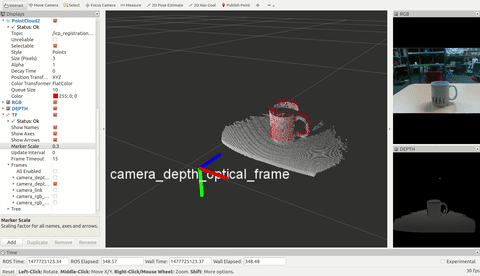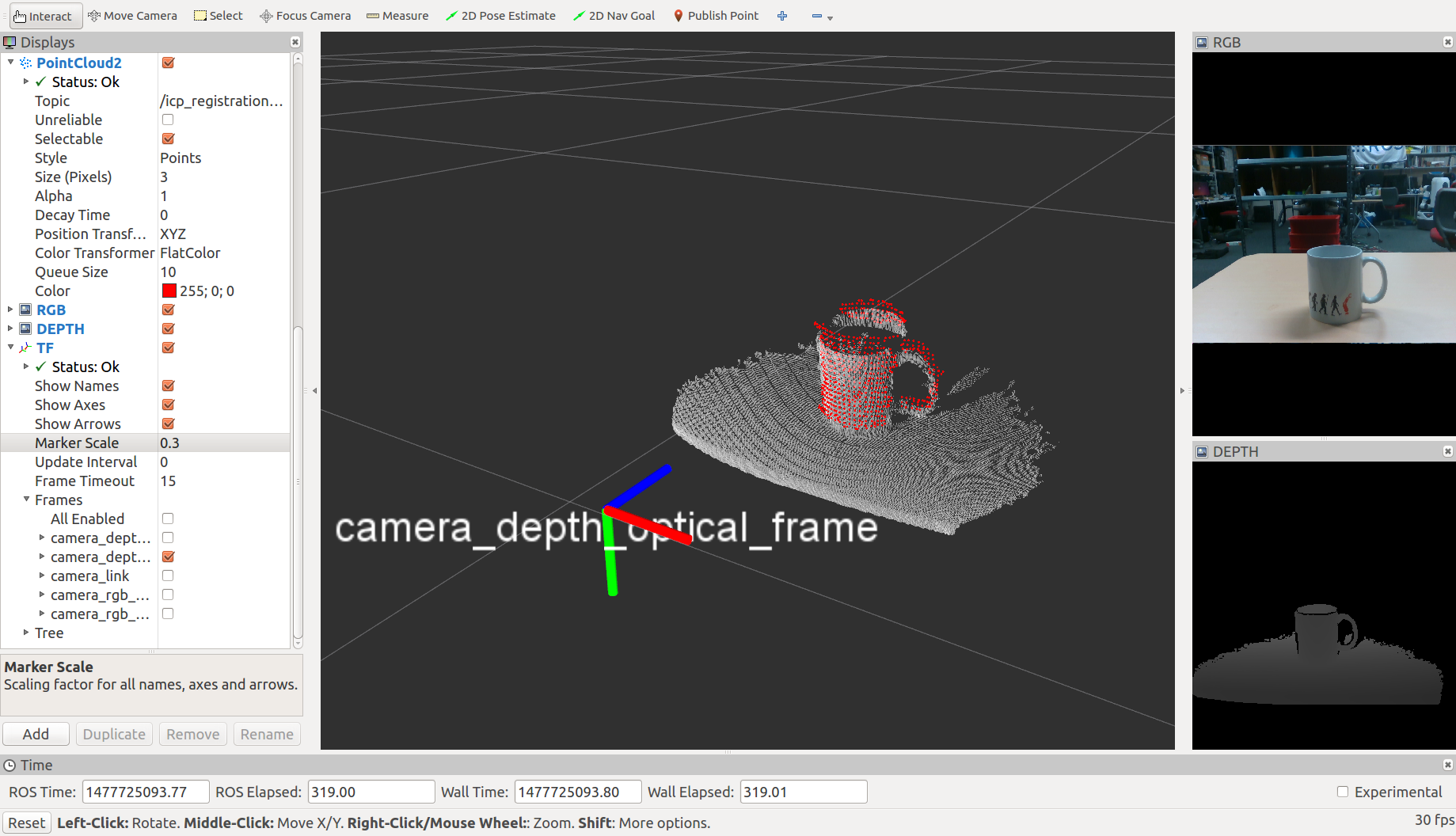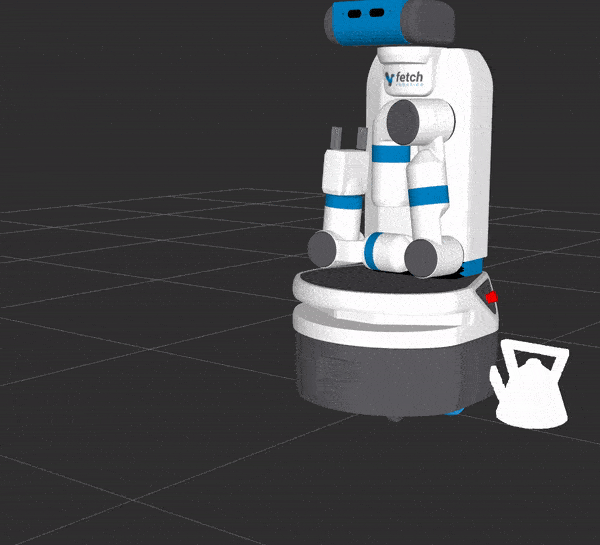# ICPRegistration¶sample_gifsample

Register two pointclouds based on icp like registration technique.

## Subscribing Topics¶

• ~input (sensor_msgs/PointCloud2)

Target pointcloud.

• ~input/camera_info (sensor_msgs/CameraInfo)

Camera info. This topic is always subscribed but needed only when ~correspondence_algorithm == Projective (1).

• ~input_reference (sensor_msgs/PointCloud2)

Reference pointcloud. frame_id of this pointlcoud is ignored.

This topic is subscribed only when ~synchronize_reference is false. Only one of ~input_reference and ~input_reference_array can be used.

• ~input_reference_array (jsk_recognition_msgs/PointsArray)

Array of reference pointcloud. ICPRegistration uses the reference which provides the best fitting score.

This topic is subscribed only when ~synchronize_reference is false. Only one of ~input_reference and ~input_reference_array can be used.

• ~input_reference_add (sensor_msgs/PointCloud2)

Reference pointcloud in addition to ~input_reference or ~input_reference_array.

This topic is subscribed only when ~synchronize_reference is false.

• ~input_box (jsk_recognition_msgs/BoundingBox)

Bounding box to align pointcloud with.

This topic is subscribed only when ~synchronize_reference is false and ~align_box is true.

• ~input_offset (geometry_msgs/PoseStamped)

Offset of pose.

It will only be subscribed if ~synchronize_reference is false and ~align_box is false and ~use_offset_pose is true.

• ~reference (sensor_msgs/PointCloud2)

Reference pointcloud.

This topic is subscribed only when ~synchronize_reference is true.

## Publishing Topics¶

• ~output (sensor_msgs/PointCloud2)

Reference pointcloud aligned with target pointcloud.

• ~output_pose (geometry_msgs/PoseStamped)

Result pose of alignment.

• ~debug/source (sensor_msgs/PointCloud2)

• ~debug/target (sensor_msgs/PointCloud2)

• ~debug/result (sensor_msgs/PointCloud2)

Pointcloud for debugging.

• ~debug/flipped (sensor_msgs/PointCloud2)

This topic is advertised but not published for now.

• ~icp_result (jsk_recognition_msgs/ICPResult)

Result pose of alignment with score and ID of best fitted reference pointcloud.

• ~output/latest_time (std_msgs/Float32)

latest computation time

• ~output/average_time (std_msgs/Float32)

average computation time

• ~icp_align (jsk_recognition_msgs/ICPAlign)

Service API of registration using target and reference pointcloud.

• ~icp_service (jsk_recognition_msgs/ICPAlignWithBox)

Service API of registration using target pointcloud and bounding box.

Reference pointcloud should be stored in advance from ~input_reference, ~input_reference_array or ~input_reference_add.

## Parameters¶

### Parameters for subscribing topics¶

• ~synchronize_reference (Bool, default: false)

If true, ~input and ~reference will be subscribed with synchronization.

If false, parameter ~align_box and ~use_offset_pose are enabled. Also, ~input_reference, ~input_reference_array and ~input_reference_add will be subscribed separately.

• ~align_box (Bool, default: false)

If true, ~input and ~input_box will be subscribed with synchronization.

• ~use_offset_pose (Bool, default: false)

If ~align_box is false and ~use_offset_pose is true, ~input and ~input_offset will be subscribed with synchronization.

If both of ~align_box and ~use_offset_pose are false, ~input will be subscribed.

### Parameters for ICP alignment¶

• ~use_normal (Bool, default: false)

Use normal information in registration. In order to use this feature, reference and target pointcloud should have valid normal fields.

• ~transform_3dof (Bool, default: false)

Add constraint to transform estimation on 3D (1D rotation + 2D translation) from header frame of input cloud. See TfTransformCloud to change header frame of point cloud.icp_2d

Parameters below can be changed by dynamic_reconfigure.

• ~algorithm (Int, default: 0)

Should be one of ICP (0), GICP (1) or NDT (2).

• ~correspondence_algorithm (Int, default: 0)

Should be one of NN (0) or Projective (1).

• ~max_iteration (Int, default: 100)

Maximum iterations of ICP alignment.

• ~correspondence_distance (Float, default: 10)

Maximum correspondence distance in meters.

• ~transform_epsilon (Float, default: 1e-9)

Maximum allowable difference between two consecutive transformations for an optimization to be considered as having converged to the final solution.

• ~euclidean_fittness_epsilon (Float, default: 0.01)

Maximum allowed Euclidean error between two consecutive steps in the ICP loop, before the algorithm is considered to have converged.

• ~ransac_iterations (Int, default: 1000)

Number of iterations RANSAC should run for.

• ~ransac_outlier_threshold (Float, default: 0.05)

Outlier distance threshold for the internal RANSAC outlier rejection loop.

• ~rotation_epsilon (Float, default: 2e-3)

Maximum allowable difference between two consecutive rotations for an optimization to be considered as having converged to the final solution.

This parameter is used only when ~algorithm is GICP (1).

• ~correspondence_randomness (Int, default: 20)

Number of neighbors used when selecting a point neighborhood to compute covariances.

This parameter is used only when ~algorithm is GICP (1).

• ~maximum_optimizer_iterations (Int, default: 20)

Maximum number of iterations at the optimization step.

This parameter is used only when ~algorithm is GICP (1).

• ~ndt_resolution (Float, default: 1.0)

• ~ndt_step_size (Float, default: 0.05)

• ~ndt_outlier_ratio (Float, default: 0.35)

These parameters are not used for now.

### Other parameters¶

• ~use_flipped_initial_pose (Bool, default: true)

Whether to consider flipped initial pose.

## Sample Launch¶

roslaunch jsk_pcl_ros sample_icp_registration.launch